Back to Adaptive Vision Library website

You are here: Start » Function Reference » Image Point Transforms

# Image Point Transforms

Select a function from the list below.

IconNameDescription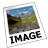AbsoluteValueImageTransforms pixel values to their absolute values pixel by pixel.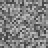AddNoiseToImageAdds random noise to the image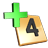AddToImageAdds a scalar value to each pixel.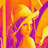ColorizeImageShows a monochromatic image in false colors.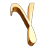CorrectGammaImage enhancement for human perception. For computer vision consider LogarithmImage.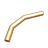CropPixelsSets pixels below the low value to the low value and above the high value to the high value.DivideImageDivides each pixel by a scalar value.InvertImageApplies numeric inversion (1/x) of the pixel values.LogarithmImageTransforms an image in such a way that a quotient on the input image becomes a difference on the output image. This can be useful for dealing with variable illumination.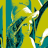LUTTransformImageChanges pixel values for data stored in array.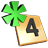MultiplyImageMultiplies each pixel by a scalar value.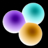NegateImageReverts the pixel value range (unsigned) or applies numeric negation (signed).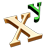PowerImageExponentiates each pixel to the given power.ReplacePixelsSubstitutes pixels having the specified value with a new value.ResaturateImageSets pixels below the low value to minimum, above the high value to maximum, and interpolates the rest.RescalePixelsApplies linear transformation to pixel values.SquareImageRaises pixel values to the second power pixel by pixel.SquareRootImageTransforms pixel values to their square roots pixel by pixel.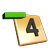SubtractFromImageSubtracts a scalar value from each pixel.

Select Filter Equivalent below.

IconNameDescriptionAvsFilter_CorrectGammaImage enhancement for human perception. For computer vision consider LogarithmImage.AvsFilter_LogarithmImageTransforms an image in such a way that a quotient on the input image becomes a difference on the output image. This can be useful for dealing with variable illumination.AvsFilter_PowerImageExponentiates each pixel to the given power.